VLOOKUP 是大家最常用的查询函数，咱先说一下VLOOKUP 的语法。

VLOOKUP (lookup_value, table_array, col_index_num, [range_lookup])

lookup_value：找什么（查找值）
table_array：哪里找（查找区域）
col_index_num：第几列（返回值在查找区域的第几列）
range_lookup：精确还是模糊（0 或 1，默认为 1）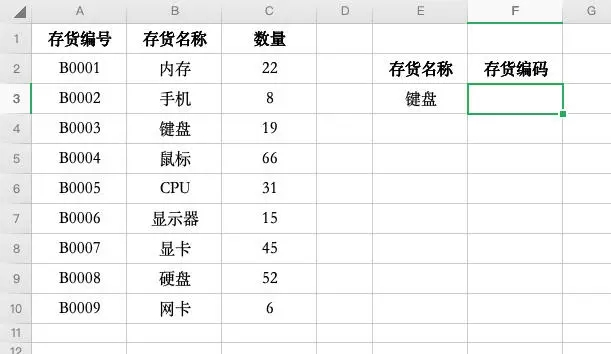1.LOOKUP
=LOOKUP(1,0/(E3=B2:B10),A2:A10)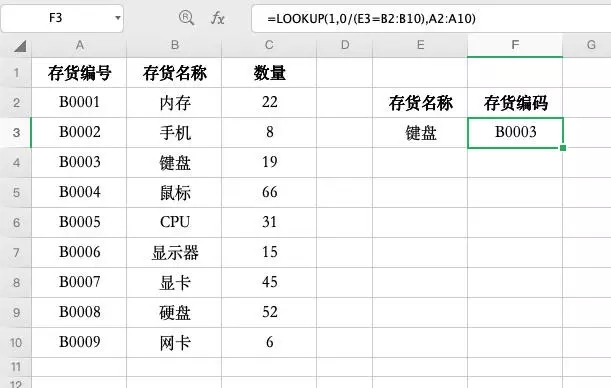2.INDEX + MATCH
=INDEX(A2:A10,MATCH(E3,B2:B10,0))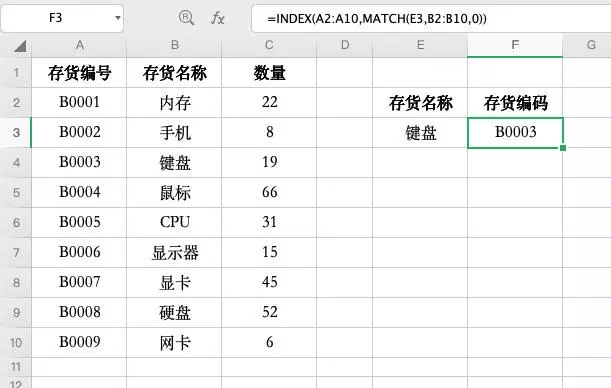3.INDIRECT + MATCH
=INDIRECT("A"&MATCH(E3,B2:B10,0)+1)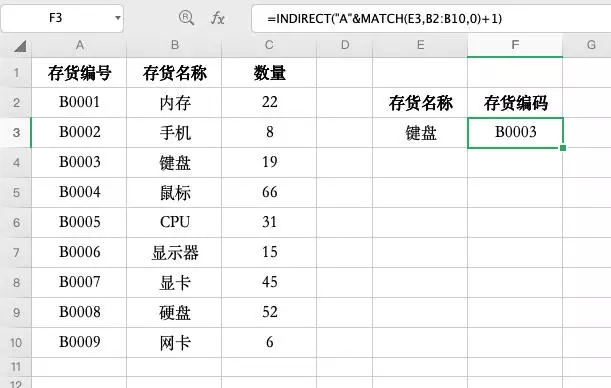INDIRECT 函数的作用是将文本转换为真正可以使用的公式。比如在单元格里输入 "=A8"，回车之后你只能看到 =A8 这几个字符，而不是 A8 这个单元格的内容 B0007

4.OFFSET + MATCH
=OFFSET(A1,MATCH(E3,B2:B10,0),)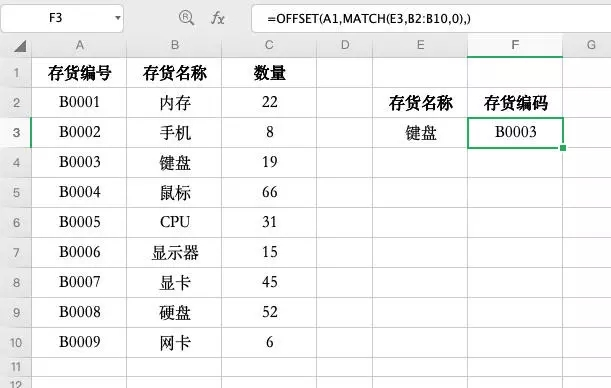OFFSET 函数是将基点单元格进行移动的函数，可以返回移动后单元格的值。它有 3 个必填参数：

5.VLOOKUP + IF
=VLOOKUP(E3,IF({1,0},B2:B10,A2:A10),2,0)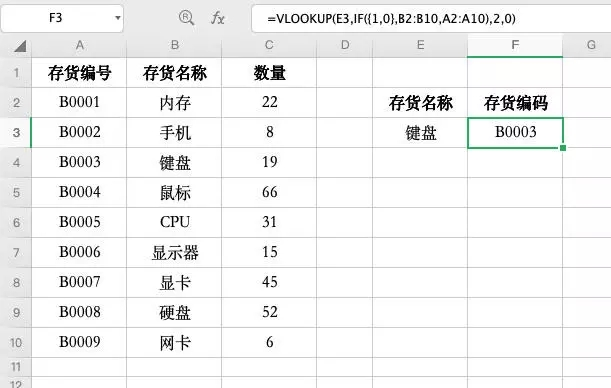6. VLOOKUP + CHOOSE
=VLOOKUP(E3,CHOOSE({1,2},B2:B10,A2:A10),2,0)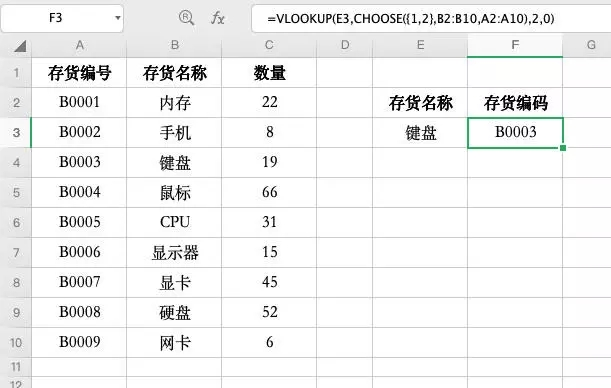Last modification：October 23, 2019
If you think my article is useful to you, please feel free to appreciate